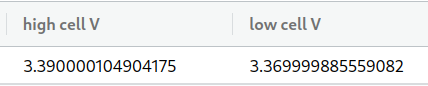# Data Bucket shows more decimals than serial monitor

There are a few discussions on here about limiting the number of decimal places displayed in data buckets. Alvarolb mentioned it could be added as an option on the web UI. That would be a good feature. (So would being able to manually sort the columns, or to preserve the order they are sent in the device write call.)

The advice is to do the rounding or truncating before sending the data. I’m doing that, using an Arduino, and the serial monitor shows the expected results, but the data bucket appears to make up additional digits?

Here’s the declaration, initialized to a missing value marker. (The multiplication has to do with how the generating device sends it.)

``````  long cell_voltage_high = -99 * 10000; // (hC) high cell voltage - multiplied by 10000
long cell_voltage_low = -99 * 10000;  // (lC) low cell voltage - multiplied by 10000
``````

I receive the data, assign it to those two variables and then include it in data to send to the bucket. In the data definition, I use the a rounding function to round to two decimal points, and reuse that function in a Serial.print call:

``````  data["high cell V"] = round_to_dp(cell_voltage_high * 0.0001, 2);
Serial.print("high cell V: ");
Serial.println(round_to_dp(cell_voltage_high * 0.0001, 2));

data["low cell V"] = round_to_dp(cell_voltage_low * 0.0001, 2);
Serial.print("low cell V: ");
Serial.println(round_to_dp(cell_voltage_low * 0.0001, 2));
``````

The serial monitor says:

``````high cell V: 3.39
low cell V: 3.37
``````

As expected, while the data bucket for that piece of data has many more digits. (The system only runs through the loop once in between 15 minute sleep sessions, so it’s easy to line up a batch of serial output with an individual row in the bucket.)